American Journal of Applied Mathematics
Volume 3, Issue 6, December 2015, Pages: 256-258

Duplication of a Cube

Lorna A. Willis

Department of Physics, Faculty of Science and Technology, University of the West Indies, Mona Campus, Jamaica, West Indies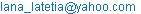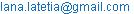Lorna A. Willis. Duplication of a Cube. American Journal of Applied Mathematics. Vol. 3, No. 6, 2015, pp. 256-258. doi: 10.11648/j.ajam.20150306.13

Abstract: A cube is a hexahedron of six identical squares. Duplication of a cube; or the Delian Problem, means starting with a cube of edge e, having a volume if v3 then proceeding to replace it by another cube of edge k, having volume, 2e3. To construct the replacement cube requires the construction of k = e 3√2. Not until the 19thCentury was it proved that there was no possible geometric construction for k= e3√ 2. The solution to this problem is to bypass the impossible and deal with the possible by starting with a range of cubes of exactly known edges, en, and their corresponding exactly known volumes, Vn, and then establishing graphically the relationship between en and Vn, to produce a practical tool. Using this practical tool in conjunction with the well established problem solving technique of working backwards, any exact value of e and its corresponding value of volume V are determined by compass extent. A determination is made of the volume of the replacement cube- the duplication- by repeating in adjoining sequence, this compass extent twice on the volume axis. Identification of the corresponding value of edge e, on the graph gives the value of k, the edge of the replacement cube. With compass extent of value, k, the cube that duplicates the original is constructed.

Keywords: Exact, Practical Tool, Working Backwards

Contents

1. Introduction

There exists a list of established problem solving techniques inclusive of one referred to as working backwards, that is starting with the known working in reverse to determine the unknown on what is desired. This technique is applied to the Duplication of a Cube or the Delian Problem. A cube is described as a hexahedron of six identical squares. Duplication of any cube means starting with an original cube of edge, e,  having volume e3. Then proceeding to replace it by another cube of edge k; having volume, 2e3. The solution to the construction of the replacement cube requires the construction of k = e 3√2. History tells us that "Not until the 19th Century was it proved that there was no possible geometric construction for k = e3√2.

However, rather than give up hope for a solution recourse is made to the working backwards technique by starting with a range of cubes of exactly known edges, en, and their corresponding exactly known volumes, Vn, and then on a Cartesian Coordinate System where the scale is an arbitrarily chosen Arithmetic Progression using the values of e as abscissa and the value of Vn as ordinate plot matching ordered pairs (e1, V1), (e2, V2), ……..(en, Vn) to obtain a graphical relationship between en and Vn which is both qualitative and quantitative in nature, and thus produce a practical tool.

To use this tool any value of e is chosen on the e-axis and the corresponding V value is identified as the volume of the original cube. The volume of the replacement cube- the duplication cube- is calculated as twice the volume of the original cube.

This value of V is used to identify its corresponding value of e which is the value of k, the edge of the replacement cube. With compass extent of value k the cube that duplicates the original is constructed.

2. Definitions, Relations and Constructions

The word, duplication in everyday use means producing exactly like another. However, in relation to the ancient geometric construction problem called the Duplication of a Cube, the phrase duplication of a cube means starting with a cube of edge e, having volume V = e3, then proceeding to replace it by another cube of edge k, having volume  Vr= 2V = 2e3= k3.

To construct the replacement cube requires the construction of k = e3√2.

The Oxford Mathematics Study Dictionary, by Frank Tapson, March 1999, defines a cube as’ a regular hexahedron and all its faces are squares". A general cube is shown in Figure 1.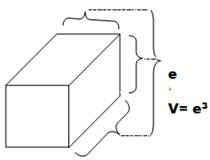Figure 1. A general cube.

A cube with edge k, and volume V= 2e3 is shown in Figure 2.

This is not a construction, just relational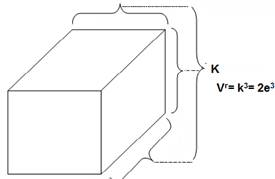Figure 2. Cube of edge K, volume Vn.

Recall history tells us that- "….there is no possible geometric construction for k = e3√2". It is acknowledged in real life that there are many ways to obtain a desired outcome. So, in this paper, the determination of k will be made using an historically different approach.

A start is made with the known. A range of cubes of known edges, en, and known corresponding volumes, Vn, are chosen as shown in Table 1, then illustrated in Figure 3.

Table 1. Relationship of ordered pairs (en, Vn).

 Edge, * en Volume, Vn 1 1 2 8 3 27 4 64 5 125 6 216 7 343 8 512 9 729 10 1000

*Size of unit arbitrarily chosen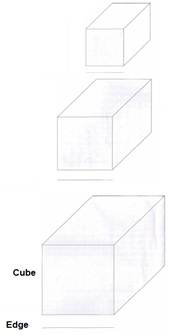Figure 3. Cubes of edge, en and Volume, Vn.

A graph is drawn of ordered pairs, (en, Vn), as shown in Figure 4. This graph is a quantification of the relationship between edge and volume of any given cube. This is the practical tool for the duplication of any cube.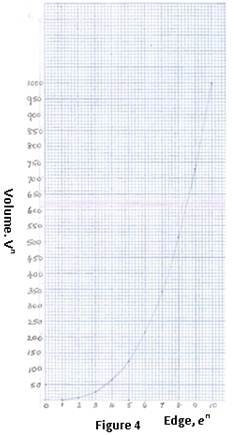Figure 4. Graph illustrating ordered pairs (en, Vn).

To use, choose a compass extent, e', then identify its corresponding volume V'. This is the volume of the starter cube. Use the compass extent corresponding to the volume V' in a twice repeat adjoining sequence, with no gap, to obtain the replacement volume, Vr= 2V', as shown in Figure 5.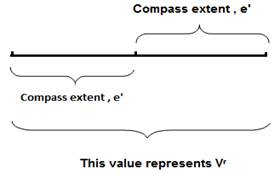Figure 5. Use of compass extent, e'.

Use this value of volume to identify the corresponding value of the edge, k. With this compass extent construct the required duplication cube as shown in Figure 3.

We know that we can construct a line with lengths of rational values, or exactly known magnitudes. We cannot construct a line from an irrational number, its magnitude. For example; we can construct a line of value 1 unit (arbitrarily chosen) and another perpendicular to it of value 2 units (of same scale). Consequently a line of value √5, which is irrational, is constructed indirectly as shown in Figure 6. So there are ways of getting around that which is difficult or problematic.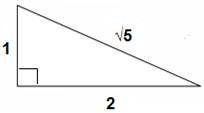Figure 6. Construction of an irrational value.

3. Conclusions

·     History does not have a monopoly on the determination of what is possible or not;

·     From the perspective of geometric construction, an irrational value of a parameter is no handicap; relationship can and does give us options

·     There is always a way to think differently, yet logically;

·     The graph itself is an array of points each of which is a geometric construction, is undeniably a valuable practical tool in geometric construction allowing us to move from one construction to another;

·     Working backwards is a valid approach to problem solving.

·     When a problem of longstanding is solved, there is a renewed confidence in reviewing positively issues that are troubling.

References

2. Tapson, Frank, "Oxford Mathematics Study Dictionary", p.46, Oxford University Press, 2006.
6. Claphan, Christopher, "The concise Oxford Dictionary of Mathematics Oxford Reference", p. 51 Oxford University Press, New York, 1990.
7. Edited by Iyanaga, Shokichi; Kawada, Yukiyosi (translated by Mathematical Society of Japan, American Mathematical Society) "Encyclopedic Dictionary of Mathematics: Mathematical Society of Japan" p. 588 mit press, Cambridge 1968.
10. "Duplication of a Cube" (2014, November 19) p.28 retrieved from http://www-math.ucdenver.edu/~wcherowi/courses/m3210/lecchap5.pdf
11. New Encyclopedia Britannica, Vol 23 Macropedia-Knowledge in Depth. Founded 1768 15th Edition, pp.581, 583 Inc. Jacob E. Safra, Chairman of the board Jorge Aguillar-Cuiz, President. London/New Delhi/Paris/Seoul/Sydney/Taipei/Tokyo © 2005.
12. Boyer,Carl B, Collier’s Encyclopedia, Vol 7 p. 160 Maxwell Macmillan Publishing Group, Copyright © 1992.
13. Rosenberg, Alex, Collier’s Encyclopedia, Vol 1 p. 543 Maxwell Macmillan Publishing Group, Copyright © 1992.

 Contents 1. 2. 3.
Article ToolsAbstractPDF(194K)